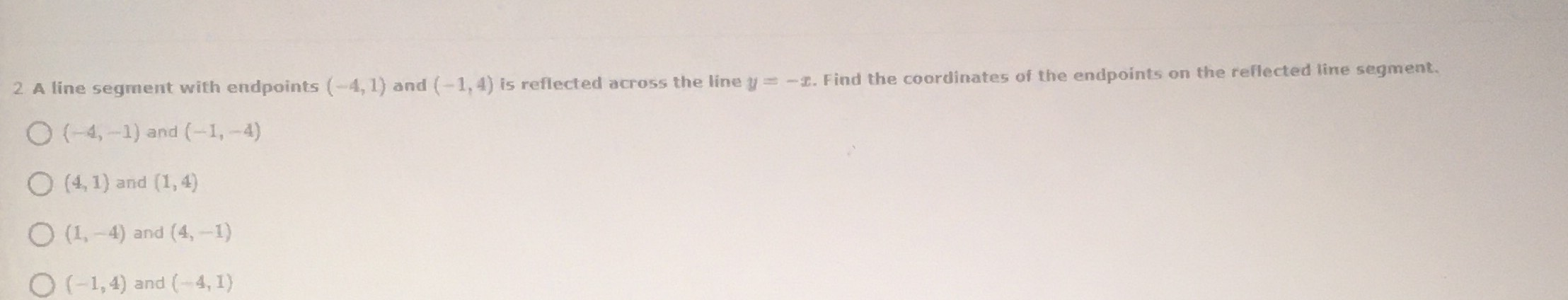### ¿Todavía tienes preguntas de matemáticas?

Pregunte a nuestros tutores expertos
Algebra
PreguntaA line segment with endpoints $$( - 4,1 )$$ and $$( - 1,4 )$$ is reflected across the line $$y = - x$$ . Find the coordinates of the endpoints on the reflected line segment.

A. $$( - 4 , - 1 )$$ and $$( - 1 , - 4 )$$

B. $$( 4,1 )$$ and $$( 1,4 )$$

C. $$( 1 , - 4 )$$ and $$( 4 , - 1 )$$

D. $$( - 1,4 )$$ and $$( - 4,1 )$$